# Math in Focus Grade 5 Chapter 2 Practice 2 Answer Key Multiplying by Tens, Hundreds, or Thousands

Go through the Math in Focus Grade 5 Workbook Answer Key Chapter 2 Practice 2 Multiplying by Tens, Hundreds, or Thousands to finish your assignments.

## Math in Focus Grade 5 Chapter 2 Practice 2 Answer Key Multiplying by Tens, Hundreds, or Thousands

Multiply.

Question 1.
47 × 10 = ___
47 X 10 = 470,

Explanation:
Given 47 multiplied by 10 we get zero at units place and
we increment towards left side as shown,
so 47 X 10 = 470.

Question 2.
38 × 10 = ___
38 X 10 = 380,

Explanation:
Given 38 multiplied by 10 we get zero at units place and
we increment towards left side as shown,
so 38 X 10 = 380.

Question 3.
109 × 10 = ___
109 X 10 = 1,090,

Explanation:
Given 109 multiplied by 10 we get zero at units place and
we increment towards left side as shown,
so 109 X 10 = 1,090.

Question 4.
521 × 10 = ___
521 X 10 = 5,210,

Explanation:
Given 521 multiplied by 10 we get zero at units place and
we increment towards left side as shown,
so 521 X 10 = 5,210.

Question 5.
7,140 × 10 = ___
7,140 X 10 = 71,400,

Explanation:
Given 7,140 multiplied by 10 we get zero at units place and
we increment towards left side as shown,
so 7,140 X 10 = 71,400.

Question 6.
1,503 × 10 = ___
1,503 X 10 = 15,030,

Explanation:
Given 1,503 multiplied by 10 we get zero at units place and
we increment towards left side as shown,
so 1,503 X 10 = 15,030.

Question 7.
3,702 × 10 = ___
3,702 X 10 = 37,020,

Explanation:
Given 3,702 multiplied by 10 we get zero at units place and
we increment towards left side as shown,
so 3,702 X 10 = 37,020.

Question 8.
9,342 × 10 = ___
9,342 X 10 = 93,420,

Explanation:
Given 9,342 multiplied by 10 we get zero at units place and
we increment towards left side as shown,
so 9,342 X 10 = 93,420.

Find the missing factors.

Question 9.
96 × __ = 960
96 X __10__ = 960,

Explanation:
As given 96 X _____ = 960 to find missing factors we divide
960 by 96 we get 10, so answer is 96 X __10 ___ = 960.

Question 10.
___ × 10 = 700
__70 __ X 10 = 700,

Explanation:
As given  _____ X 10 = 700 to find missing factors we divide
700 by 10 we get 70, so answer is  __70 _ X 10 = 700.

Question 11.
514 × __ = 5,140
514 X __10 __= 5,140,

Explanation:
As given 514 X _____ = 5,140 to find missing factors we divide
5,140 by 514 we get 10, so answer is 514 X __10 ___ = 5,140.

Question 12.
___ × 10 = 5,000
__500 __ X 10 = 5,000,

Explanation:
As given  _____ X 10 = 5,000 to find missing factors we divide
5,000 by 10 we get 500, so answer is  __500_ X 10 = 5,000.

Question 13.
308 × ___ = 3,080
308 X __10_ = 3,080,

Explanation:
As given 308 X _____ = 3,080 to find missing factors we divide
308 by 3,080 we get 10, so answer is 308 X __10 ___ = 3,080.

Question 14.
___ × 10 = 4,020
__402__ X 10 = 4,020,

Explanation:
As given _____ X 10 = 4,020 to find missing factors we divide
4,020 by 10 we get 402, so answer is __402 ___ X 10 = 4,020.

Question 15.
2,096 × ___ = 20,960
2,096 X __10 ___ = 20,960,

Explanation:
As given 2,096 X _____ = 20,960 to find missing factors we divide
20,960 by 2,096 we get 10, so answer is 2,096 X __10 ___ = 20,096.

Question 16.
___ × 10 = 91,760
__9,176__ X 10 = 91,760,

Explanation:
As given _____ X 10 = 91,760 to find missing factors we divide
91,760 by 10 we get 9,176, so answer is __9,176 ___ X 10 = 91,760.

Complete.

Example.

65 × 40 = (65 × 4) × 10
= 260 × 10
= 2,600
65 X 40 = 2,600,

Explanation:
Given 65 X 40  as 40 can be divided as 4 X 10,
So (65 X _4_) X 10,
__260__ X 10,
_2,600_,
therefore 65 X 40 = 2,600.

Question 17.
39 × 30
= (39 × _3__) × 10
= _117_ × 10
= _1,170___
39 X 30 = 1,170,

Explanation:
Given 39 X 30 as 30 can be divided as 3 X 10,
So (39 X _3_) X 10,
__117__ X 10,
_1,170_,
therefore 39 X 30 = 1,170.

Question 18.
143 × 90
= (143 × _9_) × __10__
= __1,287__ × __10__
= _12,870___
143 X 90 = 12,870,

Explanation:
Given 143 X 90 as 90 can be divided as 9 X 10,
So (143 X _9_) X 10,
__1,287__ X 10,
_12,870_,
therefore 143 X 90 = 12,870.

Question 19.
360 × 30
= (360 × _3__) × __10__
= _1,080__ × __10__
= __10,800__
360 X 30 = 10,800,

Explanation:
Given 360 X 3 as 30 can be divided as 3 X 10,
So(360 X _3_) X 10,
__1,080__ X 10,
_10,800_,
therefore 360 X 30 = 10,800.

Question 20.
285 × 80
= (285 × _8_) × _10__
= _2,280___ × _10__
= _22,800__
285 X 80 = 22,800,

Explanation:
Given 285 X 80 as 80 can be divided as 8 X 10,
So(285 X _8_) X 10,
__2,280__ X 10,
_22,800_,
therefore 39 X 30 = 1,170.

Multiply.

Question 21.
7 × 1,000 = ___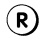7,000,

Explanation:
Given to find multiplication of 7 with thousand we get
7,000.

Question 22.
86 × 100 = ___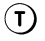8,600Explanation:
Given to find multiplication of 86 with hundred we get
8,600.

Question 23.
70 × 1,000 = ___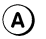70,000,

Explanation:
Given to find multiplication of 70 with thousand we get
70,000.

Question 24.
95 × 100 = __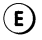9,500,
Explanation:
Given to find multiplication of 9,500 with hundred we get
9,500.

Question 25.
400 × 1,000 = ____4,00,000_____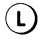4,00,000,

Explanation:
Given to find multiplication of 400 with thousand we get
4,00,000.

Question 26.
217 × 100 = ___21,700_____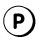21,700,

Explanation:
Given to find multiplication of 217 with hundred we get
217 X 100 = 21,700.

Question 27.
726 × 1,000 = ___72,60,000______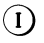72,60,000,

Explanation:
Given to find multiplication of 726 with thousand we get
72,60,000.

Question 28.
803 × 100 = _____80,300____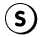80,300,

Explanation:
Given to find multiplication of 803 with hundred we get
80,300.

Question 29.
8,032 × 1,000 = ____80,32,000_______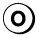80,32,000,

Explanation:
Given to find multiplication of 8,032 with thousand we get
80,32,000.

Question 30.
3,810 × 100 = ___3,81,000______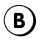3,81,000,

Explanation:
Given to find multiplication of 3,810 with hundred we get
3,81,000.

Question 31.
3,936 × 1,000 = ___39,36,000________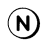39,36,000,

Explanation:
Given to find multiplication of 3,936 with thousand we get
39,36,000.

What cat has long, fine hair, and a snubbed nose?
Write the letters that match the answers below to find out.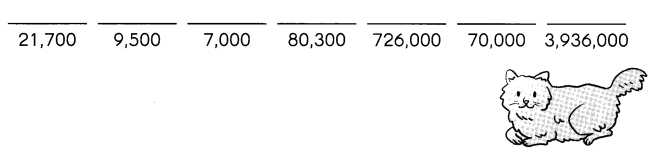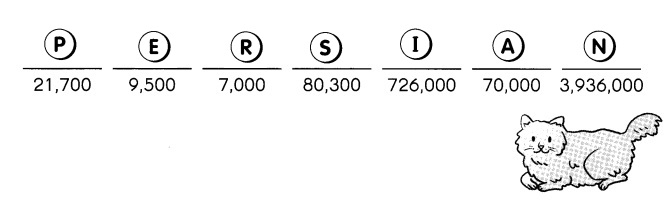PERSIAN cat has long, fine hair and a snubbed nose,

Explanation:
Persian cat has long, fine hair, and a snubbed nose,
the letters that match the answers above are written above as
P – 21,700,
E – 9,500,
R – 7,000,
S – 80,300,
I – 7,26,000,
A – 70,000,
N – 3,936,000.

Find the missing factors.

Question 32.
17 × ___100____ = 1,700

17 X 100 = 1,700,

Explanation:
Given 17 X ____ = 1,700,
to find missing factor we divide 1,700 by 17 we get
1,700 ÷ 17 = 100.

Question 33.
_25__ × 1,000 = 25,000
__25__ X 1,000 = 25,000,

Explanation:
Given ____ X 1,000 = 25,000,
to find missing factor we divide 25,000 by 1,000 we get
25,000 ÷ 1,000 = 25.

Question 34.
____4,78_____ × 1,000 = 4,78,000
__4,78___ X 1,000 = 4,78,000,

Explanation:
Given ____ X 1,000 = 4,78,000,
to find missing factor we divide 4,78,000 by 1,000 we get
4,78,000 ÷ 1,000 = 4,78.

Question 35.
320 × _1,000_ = 3,20,000
320 X __1,000__ = 3,20,000,

Explanation:
Given 320 X __1,000___ = 3,20,000,
to find missing factor we divide 3,20,000 by 320 we get
3,20,000 ÷ 320 = 1,000.

Question 36.
1,315 × ____100____ = 131,500
1,315  X __100___ = 131,500,

Explanation:
Given 1,315 X _ 100_ = 131,500
to find missing factor we divide 131,500 by 1,315 we get
131,500 ÷ 1,315 = 100.

Question 37.
_2,662__ × 1,000 = 2,662,000
__2,662__ X 1,000 = 2,662,000,

Explanation:
Given ____ X 1,000 = 2,662,000,
to find missing factor we divide 2,662,000 by 1,000 we get
2,662,000 ÷ 1,000 = 2,662.

Question 38.
4,668 × ___100___ = 4,66,800
4,668  X __100__ = 4,66,800,

Explanation:
Given 4,668 X _ 100_ = 4,66,800
to find missing factor we divide 4,668 by 100 we get
4,66,800 ÷ 4,668 = 100.

Question 39
___25_____ × 1,000 = 25,000
__25___ X 1,000 = 25,000,

Explanation:
Given ____ X 1,000 = 25,000,
to find missing factor we divide 25,000 by 1,000 we get
25,000 ÷ 1,000 = 25.

Complete.

Example.
4 × 300 = (4 × 3) × 100
= 12 × 100
= 1,200

Question 40.
12 × 500 =
= (12 × _5__) × 100
= ___60___ × 100
= __6,000__
12 X 500 = 6,000,

Explanation:
Given to find 12 X 500 so
=  (12 X __5__) X 100,
= __60__ X 100,
= __6,000_.

Question 41.
700 × 900 = (700 × __9_) × 1oo
= ___6,300___ × 100
= _6,30,000___
700 X 900 = (700 X __9__) X 100,

Explanation:
Given to find 700 X 900 so
= (700 X __9__) X 100,
= ___6,300___ X 100,
= ___ 6,30,000__.

Complete.

Question 42.
814 × 700 =
= (814 × __7_) × 100
= __5,698____ × 100
= 5,69,800
814 X 700 = 5,69,800,

Explanation:
Given to find 814 X 700 so
(814 X __7 __) X 100,
= __5,698___ X 100,
= 5,69,800.

Question 43.
5,400 × 800 =
= (5,400 × _8__) × 100
= __43,200____ × 100
= _4,320,000__
5,400 X 800= 43,200,

Explanation:
Given to find 5,400 X 800 so
= __43,200__ X 100,
= __4,320,000__.

Question 44.
5 × 7,000
= (5 × _7_) × 1,000
= ___35____ × 1,000
= __35,000__
5 X 7,000 = 35,000,

Explanation:
Given to find 5 X 7,000  so
= (5 X _7_) X 1,000,
= __35___ X 1,000,
= 35,000.

Question 45.
8 × 5,000
= (8 × _5_) × 1,000
= ___40___ × 1,000
= __40,000__
8 X 5,000 = 40,000,

Explanation:
Given to find 8 X 5,000 so
= 8 X 5 X 1,000,
= 40 X 1,000,
= 40,000.

Question 46.
12 × 3,000
= (12 × __3_) × 1,000
= _36__ × 1,000
= __36,000___
12 X 3,000 = 36,000,

Explanation:
Given to find 12 X 3,000 so
=(12 X __3__) X 1,000,
= __36__ X 1,000,
= __36,000__.

Question 47.
15 × 2,000
= (15 × __2_) × 1,000
= ___30____ × 1,000
= __30,000___
15 X 2,000 = 30,000,

Explanation:
Given to find 15 X 2,000 so
15 X 2 X 1000,
= 30 X 1,000,
= 30,000.

Question 48.
300 × 4,000
= (300 × __4_) × _1,000___
= _1,200_ × 1,000
= _12,00,000___
300 X 4,000 = 12,00,000,

Explanation:
Given to find 300 X 4,000 we get
300 x 4 X 1,000,
= 12,00 X 1,000,
=  12,00,000.

Question 49.
663 × 6,000
= (663 × _6_) × 1,000
= _3,978__ × 1,000
= _39,78,000___
663 X 6,000 =39,78,000,

Explanation:
Given to find 663 X 6,000 so
663 X 6 X 1,000,
= 3,978 X 1,000,
= 39,78,000.

Multiply.

Question 50.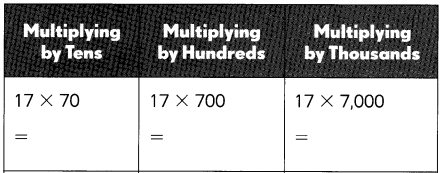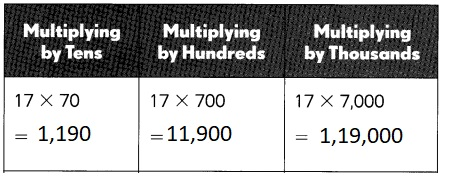Explanation:
Multiplying by Tens we get 17 X 70 as 17 X 7 X 10 = 1,190,
Multplying by Hundreds we get 17 X 700 as 17 X 7 X 100 = 11,900,
Multiplying by Thousands we get 17 X 7,000 = 17 X 7 X 1,000 = 1,19,000.

Question 51.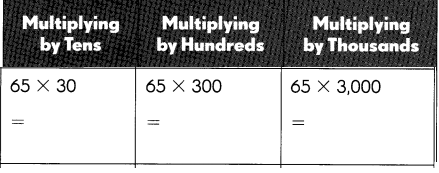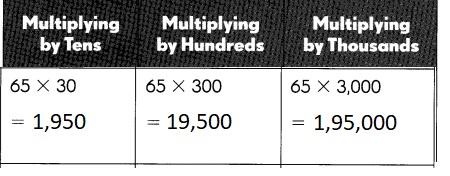Explanation:
Multiplying by Tens we get 65 X 30 as 65 X 3 X 10 = 1,950,
Multplying by Hundreds we get 65 X 300 as 65 X 3 X 100 = 19,500,
Multiplying by Thousands we get 65 X 3,000 = 65 X 3 X 1,000 = 1,95,000.

Question 52.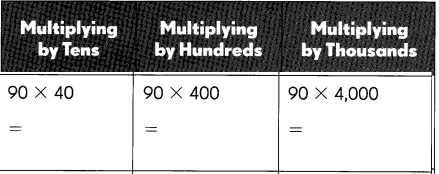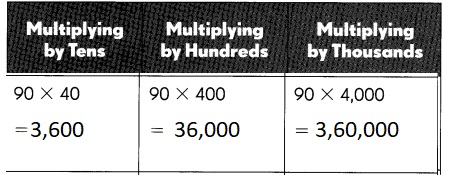Explanation:
Multiplying by Tens we get 90 X 40 as 9 X 4 X 10 = 3,600,
Multplying by Hundreds we get 90 X 400 as 90 X 4 X 100 = 36,000,
Multiplying by Thousands we get 90 X 4,000 = 90 X 4 X 1,000 = 3,60,000.

Question 53.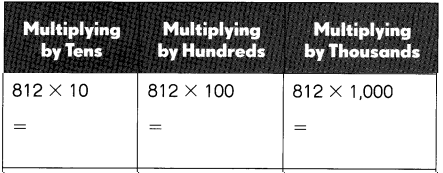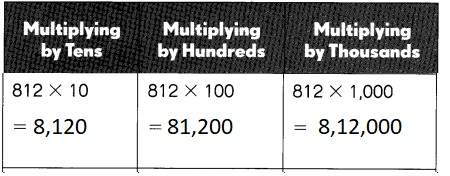Explanation:
Multiplying by Tens we get 812 X 10 as 812 X 10 = 8,120,
Multplying by Hundreds we get 812 X 100 as 812 X 100 = 81,200,
Multiplying by Thousands we get 812 X 1,000 = 812 X 1,000 = 8,12,000.

Question 54.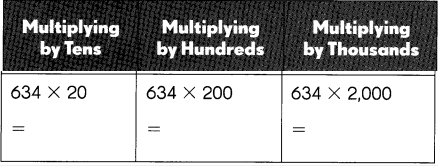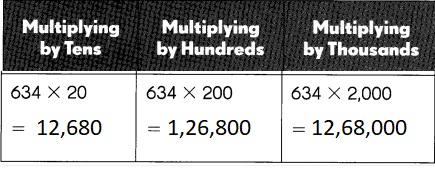Explanation:
Multiplying by Tens we get 634 X 20 as 634 X 2 X 10 = 12,680,
Multplying by Hundreds we get 634 X 200 as 634 X 2 X 100 = 1,26,800,
Multiplying by Thousands we get 634 X 200 = 634 X 2 X 1,000 = 12,68,000.

Find the missing factors.

Question 55.
31 × ___100_____ = 3,100
31 X 100 = 3,100,

Explanation:
Given to find 31 X ___ = 3,100,
the missing factor is 3,100 ÷ 31 = 100.

Question 56.
30 × ___3,000______ = 90,000
30 X ___3,000___ = 90,000,

Explanation:
Given to find 30 X _____ = 90,000,
So we divide 90,000 by 30 we get 90,000 ÷ 30 = 3,000.

Question 57.
103 × ___30_____ = 3,090
103 X _30 ___ = 3,090,

Explanation:
Given to find 103 X _____ = 3,090,
So we divide 3,090 by 103 we get 3,090 ÷ 103  = 30.

Question 58.
25 × ____200_____ = 5,000
25 X __200__ = 5,000,

Given to find 25 X _____ = 5,000,
So we divide 5,000 by 25 we get 5,000 ÷ 25 = 200.

The owner of on electronics store wants to estimate the amount she will receive from the soles of these items:

58 all-in-one printers at $219 each. 652 radio clocks at$73 each.
99 portable audio players at $217 each. 39 plasma television sets at$4,156 each.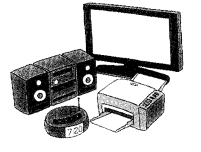The owner of the electronics store will receive $19,38,025, Explanation: Given the owner of an electronics store wants to estimate the amount she will receive from the soles of these items: 58 all-in-one printers at$219 each.
652 radio clocks at $73 each. 99 portable audio players at$217 each.
39 plasma television sets at $4,156 each are So for all-in-one printers it is 58 X$219 = $12,702, for radio clocks it is 652 X$73 = $47,596 for portable audio players it is 99 X$217 = $21,483 and for 39 plasma television sets it is 39 X$47,596 = $18,56,244. Therefore the owner will receive$12,702 + $47,596 +$21,483 + $18,56,244 =$19,38,025.

Estimate the amount she receives for each type of item by rounding to the greatest place value. Then, estimate the total amount from the sales of the items.

Question 59.
58 × $219 rounds to ___60______ ×$___220______ = $4,400 Answer:$4,400,

Explanation:
When 58 X $219 rounds to 60 X$220 we get $4,400. Question 60. 652 ×$73 rounds to ___650______ × $___70___ =$45,500
$45,500, Explanation: When 652 X$73 rounds to 650 X $70 we get$45,500.

Question 61.
99 × $217 rounds to ____100______ ×$ __220_______ = $22,000 Answer:$22,000,

Explanation:
When 99 X $217 rounds to 100 X$220 we get $22,000. Question 62. 39 ×$4156 rounds to ___40______ × $___4,160______ =$1,66,400
$1,66,400, Explanation: When 39 X$4156 rounds to 40 X $4,160 =$1,66,400.
$_4,400____ +$ __45,500___ +$__22,000___ +$1,66,400
= $_2,38,300_____ Answer:$2,38,300,
The given total estimated amount is $4,400 +$45,500 + $22,000 +$1,66,400 =